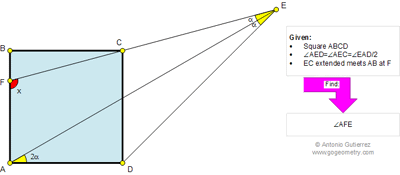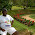## Wednesday, October 24, 2012

### Problem 818: Square, Triangle, Angles

Geometry Problem
Level: Mathematics Education, High School, Honors Geometry, College.

Click the figure below to see the complete problem 818.1.http://www.wolframalpha.com/input/?i=tg%283a%29%3Dtg%28a%29%2B1

alpha=0.30714520
x=pi/2+alpha

1.To Anonymous, problem 818: solution is not complete.

2.alpha=pi/12=15 G
x=pi/2+alpha= 105 G

I solve system of 3 equations

c=tg(a)
c+1=b*tg(3a)
c+1=(b+1)*tg(2a)

3.In the triangle AFE
AFE) = x = 180 - alpha -(90 - 2 * alpha) = 90 + alpha.
Then (DCE) = (AFE) = 90 + alpha.

Therefore:
DE = 2 AD cos(alpha) ----- (1)

In the triangle DCE :
DC/sin(2 alpha) = DE/sin(90 + alpha)
Therefore:
DE = DC / ( 2 sen(alpha) ) ----- (2).

Therefore from (1) and (2) :
2 cos(alpha) = 1/( 2 sen(alpha) ) ------ (3)

If we simplify the (3):
sin(2 alpha) = 1/2.
Therefore: alpha = 15
x = 90 + 15 = 105

4.Problem 818: Try to use elementary geometry (Euclid's Elements).

1.Geometric solution by Michael Tsourakakis
EC sectionAB=H
(q) is Circumcircle of the scare ABCD and (q) section AE=K
bisector of the angle EAD section (q) =M.
angleCAK=α=angleCEA.So, angleHKA=2α =30
From the triangle HCA : x=180-30-45=1050

2.http://1.bp.blogspot.com/-H0dtaRbb9bY/UMTFm5yQRyI/AAAAAAAAAAM/5Dco1bojssk/s1600/Untitled.jpg

5.Prof Radu Ion,Sc.Gim.Bozioru,BuzauDecember 21, 2012 at 3:36 AM

Daca notam cu P intersectia dreptelor CF si AD vom obtine:2α=m(m( in triunghiul AFP dreptunghic in A, m(
x=m(<AFE)=180-(90-α)=90+α

6.To: Michael Tsourakakis---In your solution you have implied that the ARC CKMD is split into 3 equal parts. This is not true based on your reasoning.

1.correct solution
(q) is Circumcircle of the square ABCD and (q) section AE=K
BD ,is mediator of AC and V is mediator of CE. BD section (v)=M.Then , CM is mediator of AE ,so CM is perpendicular of the AE.Beacause AC is ,diameter the circle (q) ,CK ,is perpendicular of AE and the points C,K,M , are collinear . The triangle ACE ,is isosceles.
So, angle CAE=α and angleCAD=3α=45 . Τhen,α=15
x=180-45-2α =135-30=105 degrees
http://img191.imageshack.us/img191/5597/geogebra3.png
(Michael Tsourakakis )

2.correction:
The triangle CAE is isosceles And not "the triangle ΜΑΕ is isosceles"
(Michael Tsourakakis

7.Anonymous

If we say the cut of BD with CF = P and
the cut AE with CD =R you have accept without verification that angle CPR= 2 alpha or equivalent that angle APR =90 degree .
If you can not verify one of these two saying than the solution is wrong.

1.you're right.Thanks for the correction.I'll look again
HAPPY NEW YEAR
Michael Tsourakakis

8.http://img839.imageshack.us/img839/3518/problem818.png

Draw circumcircle of triangle CDE
Note that angleDOG= 2 α and AE cut arc CD at midpoint G
And OD=AD=CD => triangle DOC is a equilateral
So 2 α= 30
In triangle AFE , we have x=180- α-(90-2 α)= 90+ α= 105 degrees

1.Excellent work Peter

9.http://www.mathematica.gr/forum/viewtopic.php?f=22&t=32386&p=149846

10.Drop a perpendicular from D to AG to meet AG at X and CF at Y. Let AG meet CD at Z.

DX is an altitude of right ∆ ADZ, hence < ZDY = DAZ = 2α.
But < DYC = 2α considering ∆GXY, so < DCY also = 90-α.
Therefore DY = DC

So DX = ½ DY = ½ DC = ½ AD
Hence ADX is a 30-60-90 ∆ and 2α = 30 and α = 15

Finally from ∆ AGF, x = 180 - (90 - 2α) - α =90 + α = 105

Sumith Peiris
Moratuwa
Sri Lanka